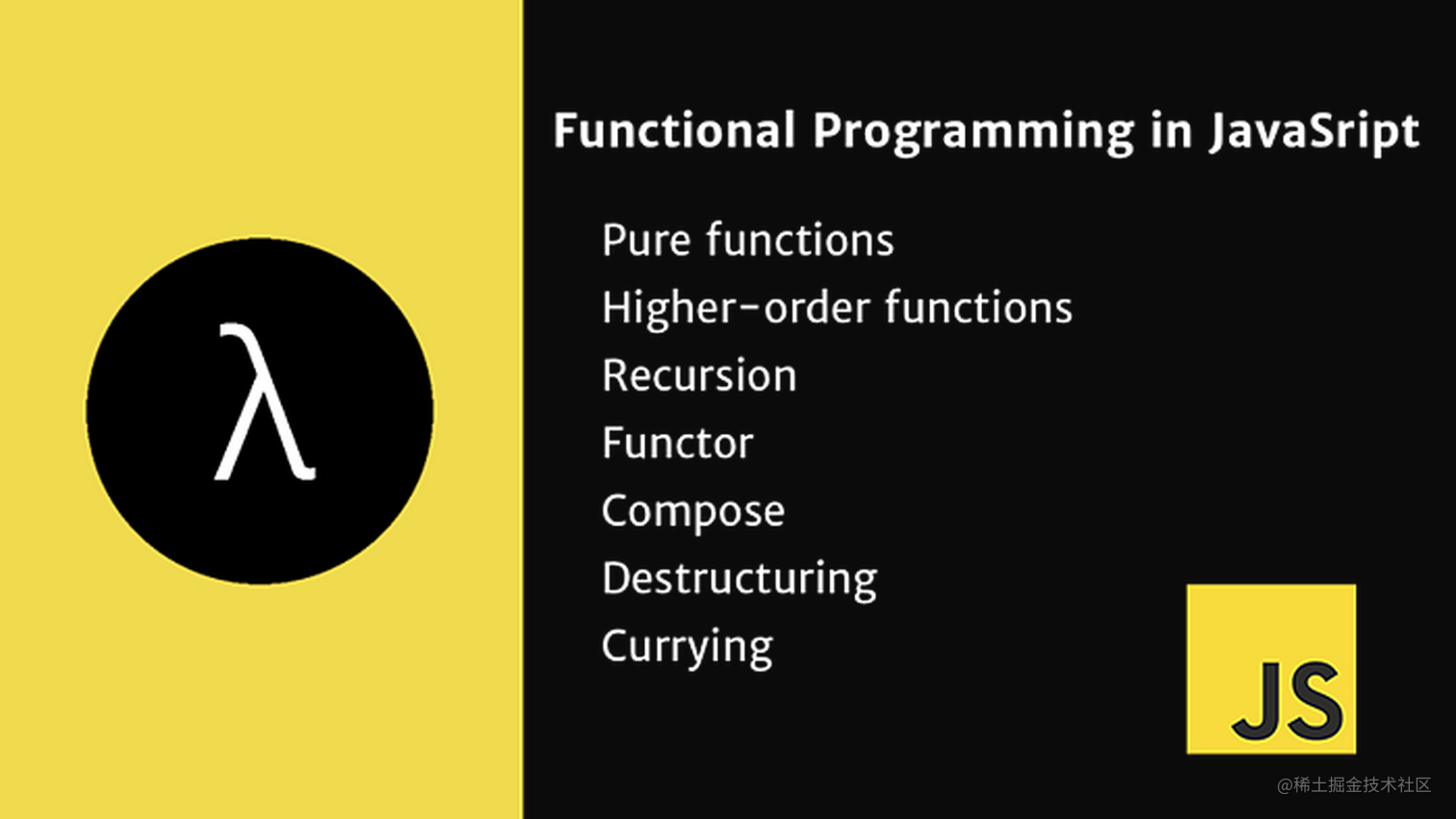# JS函数式编程&高阶函数的应用## 前言

### 函数是 JavaScript 的一等公民

• 可以被存入变量或者其他数据结构
• 可以作为函数的参数
• 可以作为函数的返回值
• 可以判断相等性

## 纯函数

``````const sum = (x, y) => x + y;
sum(1, 2) // 3

``````let arr = [1, 2, 3];
arr.slice(0,1); // 
arr.slice(0,1); // 
arr // [1, 2, 3]

arr.splice(0,1); // 
arr.splice(0,1); // 
arr // 

### 纯函数的好处 - memoize

``````const memoize = f => { // 缓存函数
let cache = {};
return a => { // 这里为了方便 就只接收一个参数了
const prevValue = cache[a];
if (prevValue) {
console.log(`cache \${prevValue}`);
return prevValue;
}
return cache[a] = f(a); // 缓存纯函数的值
}
}
const double = x =>  x * 2; // 运算函数（纯函数）
const f = memoize(double);

f(2); // 4
f(2); // cache 4

f(8); // 16
f(8); // cache 16

## 柯里化 Curry

• 可以将上述求和纯函数改成下面的写法
``````const sum = x => y => x + y;
sum(1)(2); // 3

`sum` 函数转为只接收一个参数，并返回一个函数，再次调用将得到结果。 或许有人觉得这样写纯属蛋疼，那么就举一个业务中会用到的一个例子。我们需要一个可以将金额转为千分位的函数，但是金额的单位不定，有可能是分，有可能是角等。

``````const formatMoney = (money, step) => {
let str = (money / step).toFixed(2); // 两位小数
const index = str.indexOf('.');
if (index > 3) {
const start = str.substring(0, index).replace(/\B(?=(?:\d{3})+\$)/g, ','); // 增加千分位符号
return start + str.substring(index);
};
return str;
}
formatMoney(1000000, 100); // 分 10,000.00
formatMoney(123456, 100); // 分 1,234.56
formatMoney(123456, 10); // 角 12,345.60

``````const formatMoney = step => money => {
let str = (money / step).toFixed(2); // 两位小数
const index = str.indexOf('.');
if (index > 3) {
const start = str.substring(0, index).replace(/\B(?=(?:\d{3})+\$)/g, ','); // 增加千分位符号
return start + str.substring(index);
};
return str;
}
const pennyMoney = formatMoney(100); // 单位是分
pennyMoney(1000000); // 10,000.00
pennyMoney(123456); // 1,234.56

const dimeMoney = formatMoney(10); // 单位是角
dimeMoney(1000000); // 100,000.00
dimeMoney(123456); // 12,345.60

formatMoney(1)(1000000); // 1,000,000; // 元

## 高阶函数

### 高阶函数的应用

#### 🌰 防抖函数

``````const debounce = (func, wait) => {
let timer = null;
return (...args) => {
clearTimeout(timer);
timer = setTimeout(() => {
func(...args);
}, wait)
}
}

if (window.matchMedia('(min-width:768px)')) {
}
console.log('resize');
}, 500))

#### 🌰 组合 compose

``````const compose = (...funcs) => arg => {
return funcs.reduce((val, f) => f(val), arg);
}

``````const compose = (...funcs) => arg => {
return funcs.reduce((val, f) =>{
const result = f(val);
console.log(result);
return result;
}, arg);
}

const pennyTransform = money => money / 100;
const fixedMoney = money => (+money).toFixed(2);
const thousandthMoney = str => {
const index = str.indexOf('.');
if (index > 3) {
const start = str.substring(0, index).replace(/\B(?=(?:\d{3})+\$)/g, ',');
return start + str.substring(index);
};
return str;
};

const formatMoney = compose(pennyTransform, fixedMoney, thousandthMoney);
formatMoney(1000000);

// 10000
// 10000.00
// 10,000.00

`callback` 执行数组中每个值，此函数会接收四个参数，这里我们只看用到的前两个参数：

• `accumulator` 累计器累计回调的返回值，简单理解就是上一个调用回调函数的返回值。如果是第一个次调用那么就是下面的 `initialValue` 或者 `undefined`

• `currentValue` 数组中当前正在处理的元素。我们数组内的元素都是方法，也就是这里将是当前需要执行的方法。

`initialValue` 第一次调用回调函数传的值。

``````const compose = (...funcs) => initialValue => {
return funcs.reduce((accumulator, currentValue) => {
// 第一次执行 accumulator 为传入的 initialValue

// 将 accumulator 传入 currentValue（当前需要执行的方法），
// 这里也会将函数调用的结果进行立即返回，返回的值将会在执行到下一个函数时当 accumulator 使用
// 一直到执行完最后一个函数，将最后一个函数的返回值进行返回。
return currentValue(accumulator);
}, initialValue);
}

## 最后

2020.8.25更新 在 React 中自定义 Hooks 的应用场景

• 王大冶
42分钟前• 阳树阳树
27分钟前• Lvzl
35分钟前•• 道长王jj
20小时前• Jay丶
6天前•• 童欧巴
13小时前• 翰玥
4天前• 布衣1983
13小时前
••• Cosolar
11小时前• 慢功夫
19小时前
• 德育处主任
19小时前• superZidan
10天前• ymitc
1天前•